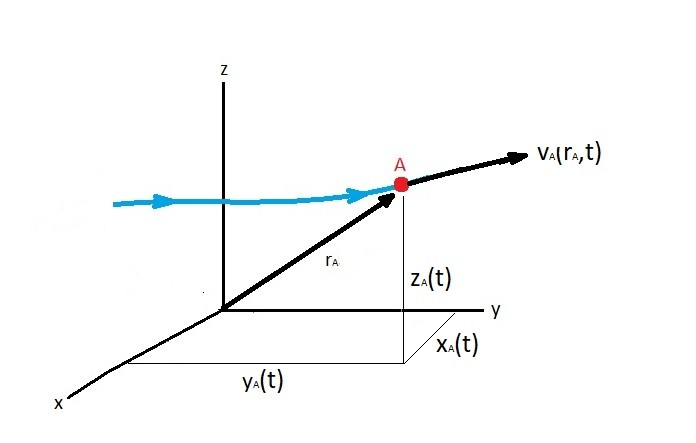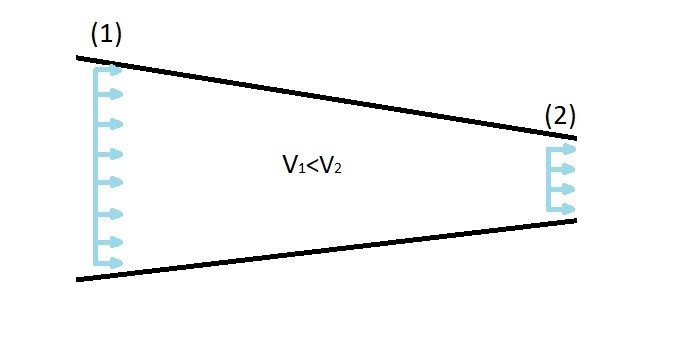# Material Derivative

To use Newton’s second law you will need derive an acceleration field.  You can use the Lagrangian method to define the acceleration field.  By using the Lagrangian method you will track individual fluid particles.  You can do this by using the material derivative.

First, let’s talk about acceleration. So when does fluid particle Acceleration occur?  One way that fluid acceleration will occur is when the flow is unsteady.  As result the fluid velocity at a given point will vary with time.  Acceleration of fluid particle will also occur when the fluid velocity changes do a change in cross-sectional area.  This type of acceleration will occur for both steady and unsteady states of flow.

### The Material Derivative

Now let’s talk about the material derivative. To derive the material derivative you will first need to consider a fluid particle that is moving along a pathline.  Refer to the image below.Let’s take a look at particle A’s velocity ($v_A$).  The particle velocity will be both a function of time and location.

(Eq 1) $v_A=v_A(r_A,t)=v_A[x_A(t), y_A(t), z_A(t),t]$

To define acceleration you would need define the velocities rate of change in respect to time.  However, as stated above, the velocity of a particle is both a function of time and location.  As a result, to derive the acceleration equation from equation 1 you will need to use the chain rule.

(Eq 2) $a_A(t)=\frac{dv_A}{dt}=\frac{∂v_A}{dt}+\frac{∂v_A}{∂x}\frac{dx_A}{dt}+\frac{∂v_A}{∂y}\frac{dy_A}{dt}+\frac{∂v_A}{∂z}\frac{dz_A}{dt}$

Equation 2 can be simplified by defining the velocity components in the following manner.  $u_a = dx_A/dt$, $ν_A=dy_A/dt$, and $w_A=dz_A/dt$.

$a_A = \frac{∂v_A}{∂t}+u_A\frac{∂v_A}{∂x}+ν_A\frac{∂v_A}{∂y}+w_A\frac{∂v_A}{∂z}$

The above equation will apply to all fluid particles.  As a result the subscript A can be removed from the equation.  This will result in equation 3.

(Eq 3) $a= \frac{∂v}{∂t}+u\frac{∂v}{∂x}+ν\frac{∂v}{∂y}+w\frac{∂v}{∂z}$

Equation 3 represent the vector equation of for the acceleration of a fluid particle.  This can be broken into the following scalar equations to obtain the acceleration of the fluid particle in the x, y, and z directions.

$a_x=\frac{∂u}{∂t}+u\frac{∂u}{∂x}+ν\frac{∂u}{∂y}+w\frac{∂u}{∂z}$

$a_y=\frac{∂ν}{∂t}+u\frac{∂ν}{∂x}+ν\frac{∂ν}{∂y}+w\frac{∂ν}{∂z}$

$a_z=\frac{∂w}{∂t}+u\frac{∂w}{∂x}+ν\frac{∂w}{∂y}+w\frac{∂w}{∂z}$

Finally, the above the equations can be written in short hand notation.

$a=\frac{Dv}{Dt}$

where the operator is

(Eq 4) $\frac{D(~)}{Dt}≡\frac{∂(~)}{∂t}+u\frac{∂(~)}{∂x}+ν\frac{∂(~)}{∂y}+w\frac{∂(~)}{∂z}$

Equation 4 represent the material derivative.  Often the material derivative will be written in shorthand notation, as seen in the equation below.

(Eq 5) $\frac{D(~)}{Dt}=\frac{∂(~)}{∂t}+(V·∇)(~)$

### Convective Effects

Again as mention above the material derivative has two parts, the time derivative and the spatial derivatives.  While the time derivative represents local acceleration, the spatial derivative represents the convective effects.  As a result the spatial derivatives are also called the convective derivatives.  The convective derivatives represents the fact that the flow properties from one point to another point can change.  An example of this would be a fluid flowing through a nozzle.  As the fluid flows through the nozzle its velocity will increase as the diameter of the nozzle decreases.  Hence an acceleration occurs on the fluid particles regardless of whether the flow is steady or unsteady.  As a result convective acceleration occurs due to a change in geometry.|